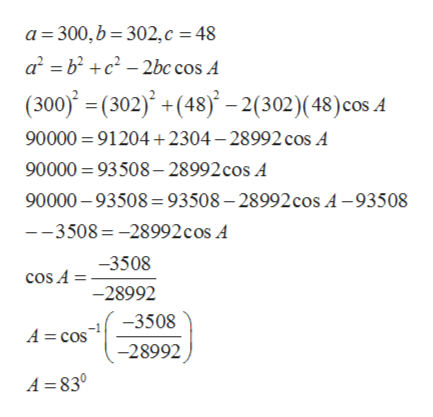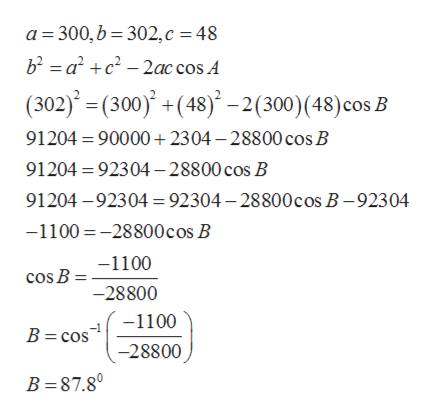# use the Law of Cosines to find the remaining side(s) and angle(s) if possible. a=300, b=302, c=48

Question
23 views

use the Law of Cosines to find the remaining side(s) and angle(s) if possible. a=300, b=302, c=48

check_circle

Step 1

use the Law of Cosines to find the remaining side(s) and angle(s) if possible. a=300, b=302, c=48

Step 2

Use cosine formula to find angle Ahelp_outlineImage Transcriptionclosea 300,b 302,c 48 a bc2bc cos A (300)= (302) +(48)-2(302)( 48) cos A 90000 91204 2304-28992 cos A 90000 93508-28992 cos A 90000-93508= 93508-28992 cos A-93508 --3508-28992 cos A -3508 cos A = -28992 -3508 A cos -28992 A =830 fullscreen
Step 3

Use cosine formula to...help_outlineImage Transcriptionclosea 300,b 302,c 48 b2 a c-2ac cos A (302)(300)+(48)-2(300)(48) cos B 91204 90000+ 2304-28800 cos B 91204 92304-28800 cos B 91204-92304 92304-28800 cos B -92304 -1100-28800cos B -1100 cos B = -28800 -1100 B = cos -28800 B 87.80 fullscreen

### Want to see the full answer?

See Solution

#### Want to see this answer and more?

Solutions are written by subject experts who are available 24/7. Questions are typically answered within 1 hour.*

See Solution
*Response times may vary by subject and question.
Tagged in

### Calculus